Test: Theory of Columns - 1

# Test: Theory of Columns - 1

Test Description

## 10 Questions MCQ Test GATE Civil Engineering (CE) 2023 Mock Test Series | Test: Theory of Columns - 1

Test: Theory of Columns - 1 for Civil Engineering (CE) 2022 is part of GATE Civil Engineering (CE) 2023 Mock Test Series preparation. The Test: Theory of Columns - 1 questions and answers have been prepared according to the Civil Engineering (CE) exam syllabus.The Test: Theory of Columns - 1 MCQs are made for Civil Engineering (CE) 2022 Exam. Find important definitions, questions, notes, meanings, examples, exercises, MCQs and online tests for Test: Theory of Columns - 1 below.
Solutions of Test: Theory of Columns - 1 questions in English are available as part of our GATE Civil Engineering (CE) 2023 Mock Test Series for Civil Engineering (CE) & Test: Theory of Columns - 1 solutions in Hindi for GATE Civil Engineering (CE) 2023 Mock Test Series course. Download more important topics, notes, lectures and mock test series for Civil Engineering (CE) Exam by signing up for free. Attempt Test: Theory of Columns - 1 | 10 questions in 30 minutes | Mock test for Civil Engineering (CE) preparation | Free important questions MCQ to study GATE Civil Engineering (CE) 2023 Mock Test Series for Civil Engineering (CE) Exam | Download free PDF with solutions
 1 Crore+ students have signed up on EduRev. Have you?
Test: Theory of Columns - 1 - Question 1

### For a circular column having its ends hinged, the slenderness ratio is 160. The l/d ratio of the column is

Detailed Solution for Test: Theory of Columns - 1 - Question 1

If radius R then radius of gyration = R/2.
Now , L ÷ (R/2)=160,
-) L ÷ (D/4)=160,
So, L/D= 40.

Test: Theory of Columns - 1 - Question 2

### For the case of slender column of length 'l’ and flexural rigidity EI built in at its base and free at the top, the Euler's buckling load is

Detailed Solution for Test: Theory of Columns - 1 - Question 2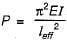For a column fixed at base and free at the top,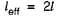∴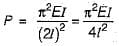Test: Theory of Columns - 1 - Question 3

### Rankine-GordonTormulafor buckling is valid for

Test: Theory of Columns - 1 - Question 4

The resultant cuts the base of a circular column of diameter ‘d’ with an eccentricity equal to one-fourth of 'd'. The ratio between the maximum compressive stress and the maximum tensile stress is

Detailed Solution for Test: Theory of Columns - 1 - Question 4

For a solid circular section, the maximum eccentricity for no tension is d/8.
The stress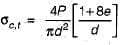for e = d/4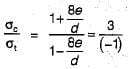The minus sign is due to opposite nature of forces.

Test: Theory of Columns - 1 - Question 5

A column of length 2.4 m area of cross-section 2,000 mm2 andmoment of inertia of Ixx = 720 x 104 mm4 and Iyy = 80 x 104 mm4 is subjected to buckling load. Both the ends of the column are fixed. What is the slenderness ratio of column?

Detailed Solution for Test: Theory of Columns - 1 - Question 5

Slenderness ratio of column is given by,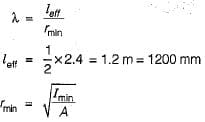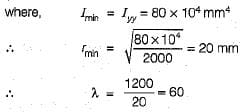Test: Theory of Columns - 1 - Question 6

A hollow circular column, has D = 100 mm, d = 80 mm. What is its radius of gyration

Detailed Solution for Test: Theory of Columns - 1 - Question 6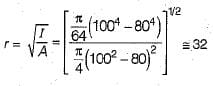Test: Theory of Columns - 1 - Question 7

If diameter of a long column is reduced by 20%, the percentage reduction in Euler’s buckling load is

Detailed Solution for Test: Theory of Columns - 1 - Question 7

Euler buckling load P is given by,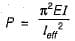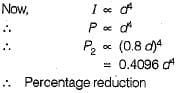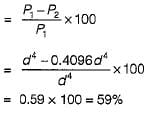Test: Theory of Columns - 1 - Question 8

A rectangular bar as shown in the figure below carries a tensile load of 1 kN as shown in the figure. Under this load the maximum value of stress over the mean value will increase by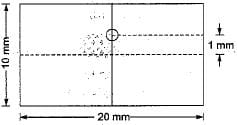Detailed Solution for Test: Theory of Columns - 1 - Question 8

Mean value of stress,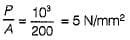Maximum intensity of stress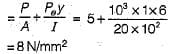∴ Increase in stress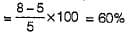Test: Theory of Columns - 1 - Question 9

A hollow circular column of internal diameter 'd' and external diameter '1.5 d’ is subjected to compressive load. The maximum distance of the point of application of load from the centre for no tension is

Detailed Solution for Test: Theory of Columns - 1 - Question 9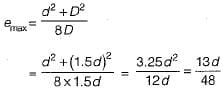Test: Theory of Columns - 1 - Question 10

Given that
PE = The crippling load given by Euler
PC = The load at failure due to direct compression
PR = The load in accordance with the Rankine's criterion of failure
PR is given by

## GATE Civil Engineering (CE) 2023 Mock Test Series

25 docs|282 tests
 Use Code STAYHOME200 and get INR 200 additional OFF Use Coupon Code
Information about Test: Theory of Columns - 1 Page
In this test you can find the Exam questions for Test: Theory of Columns - 1 solved & explained in the simplest way possible. Besides giving Questions and answers for Test: Theory of Columns - 1, EduRev gives you an ample number of Online tests for practice

## GATE Civil Engineering (CE) 2023 Mock Test Series

25 docs|282 tests